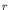Computational & Technology Resources an online resource for computational,engineering & technology publications not logged in - login Civil-Comp ProceedingsISSN 1759-3433 CCP: 83PROCEEDINGS OF THE EIGHTH INTERNATIONAL CONFERENCE ON COMPUTATIONAL STRUCTURES TECHNOLOGY Edited by: B.H.V. Topping, G. Montero and R. Montenegro Paper 262A Modified Three-Dimensional Analytical Model for Stress Prediction in Short Fibre Composites M. Pahlavanpour, A. Abedian and M. MondaliDepartment of Aerospace Engineering, Sharif University of Technology, Tehran, Iran doi:10.4203/ccp.83.262 Full Bibliographic Reference for this paper M. Pahlavanpour, A. Abedian, M. Mondali, "A Modified Three-Dimensional Analytical Model for Stress Prediction in Short Fibre Composites", in B.H.V. Topping, G. Montero, R. Montenegro, (Editors), "Proceedings of the Eighth International Conference on Computational Structures Technology", Civil-Comp Press, Stirlingshire, UK, Paper 262, 2006. doi:10.4203/ccp.83.262 Keywords: analytical modelling, short fibre composites, interface debonding, FEM. Summary Obtaining the exact elastic response of composite systems to externally applied stresses is thought to be the most important way for predicting the mechanical behavior and tailoring the properties of composites. Therefore, it is so important to know the stress distribution inside the composite and the ways of stress transfer between the fiber constituents. Over the past few decades, a variety of analytical models has been developed to investigate different stress transfer mechanisms in fiber composites. These analytical models can be roughly divided into two categories, i.e. one-dimensional models typically based on the shear-lag theory  and three-dimensional analytical models based on axisymmetric analyses [4,5,6,7]. The early 'shear-lag' analysis by Cox  is widely used for the stress transfer analysis in unidirectional continuous or short fiber composites. The solution using the shear-lag model is based on a simple differential equation relating the fiber axial stress to the interfacial shear stress. Although, the predicting capability of the shear-lag model has been improved through various modifications [2,3], the model still needs to be modified due to some drawbacks inherent with the structure of the model. These drawbacks stem mainly in an assumption that (for an axisymmetric case) the axial stress and strain in the matrix are independent of the axial and radial locations in the matrix, which has been proved to be unreasonable by many experimental and numerical investigations. Most of the interfacial problems require detailed distribution of the stresses at the interface such as the interface friction slip behavior in composites, but the one-dimensional shear-lag model can not provide this stress state. However, three-dimensional analytical models were developed based on quite different approaches and aimed mostly at the interfacial problems [4,5]. It is noted that the equilibrium states and most of the boundary and interfacial conditions are generally satisfied by most of the three-dimensional analytical models. This is because the solutions are usually based on the stress equilibrium equations, however the compatibility conditions are only partly or approximately satisfied due to different degrees of approximations and simplifications used. Therefore, because of the excessive complicated mathematical derivations involved, very limited efforts for exact solution of such problems could be found in the literature. One of the most exact and relatively simple three-dimensional analytical solutions belongs to Jiang et al. . In this model, two sets of exact displacement solutions for the matrix, i.e. the far-field solution and the transient solution, are derived. Then, using the theory of elasticity and superposition of the simplified analytical expressions, all the stress components in the matrix and the axial uniform stress component in the fiber could be found. Note that the fiber end region could also be included with the use of imaginary fiber technique. In brief, for the Jiang's model, the following assumptions were made; e.g. a perfect bond at the fiber-matrix interface exist, both fiber and matrix are isotropic, the fiber axial stress and strain components are independent of the radial position, and the radial and hoop stress components are equal at the interface. In the present study, the above assumptions due to the following reasons are changed. Along the whole fiber-matrix interface, the radial and hoop stress components are not equal and the Jiang's model does not have the capacity of solving the end and partial debonding problems (along the fiber length). In the present research work, considering the above changes in the assumptions, the modified analytical model produced the following results and conclusions which were then validated by FEM modeling. The predicting capability of Jiang's model is greatly improved. The dependency of the fiber and imaginary fiber stresses and strains to the-direction was shown and calculated. The mathematical term indicates the difference between the interfacial radial and hoop stress components is obtained. Finally, the most important aspect of the modified model is its capability of presenting analytical three-dimensional expressions for the stress field both in the fiber and matrix regions. Note that Jiang's model only predicts the stress field in the matrix and the interface regions. Also, Jiang's model is unable to calculate the stress filed in the imaginary fiber region, which is easily done using the modified model. References 1 H. L. Cox, Brit. J. Appl. Phys. 3 72-79, 1952. doi:10.1088/0508-3443/3/3/302 2 A. Kelly, W.R. Tyson, J. Mech. Phys. Solids, 329-350, 1965. doi:10.1016/0022-5096(65)90035-9 3 C.H. Hsueh, R.J. Young, X. Yang, P. F. Becher, Acta, mater. 45 (4), 1469-1476, 1997. doi:10.1016/S1359-6454(96)00262-5 4 W. Wu, I. Verpoest, J. Varna, Compos. Sci. Technol. 57, 809-819, 1997. doi:10.1016/S0266-3538(97)00050-X 5 J.A. Nairn, D.A. Mendels, Mech. Mater. 33, 335-362, 2001. doi:10.1016/S0167-6636(01)00056-4 6 Z. Jiang, J. Lian, D. Yang, S. Dong, Mater. Sci. Eng. A 248, 256-275, 1998. doi:10.1016/S0921-5093(98)00509-7 7 Z. Jiang, X. Liu, G. Li, J. Lian, Mater. Sci. Eng. A 366, 381-396, 2004. doi:10.1016/j.msea.2003.09.055 purchase the full-text of this paper (price £20) Back to top ©Civil-Comp Limited 2020 - terms & conditions